Conditions for Two Functions in the Plane to be Functionally Related

Theorem

A necessary and sufficient condition for functions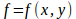and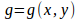to be related by the function F with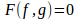is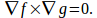Proof

Suppose thatthen using the chain rule gives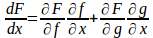and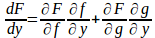(1)

Since {{partial F} over {partial f}} and {{partial F} over {partial y}} are not both zero, the equations (1) are consistent only if the determinant of the coeefient matrix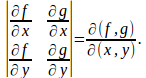But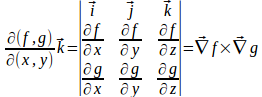so